Integration Full Chapter Explained - https://you.tube/Integration-Class-12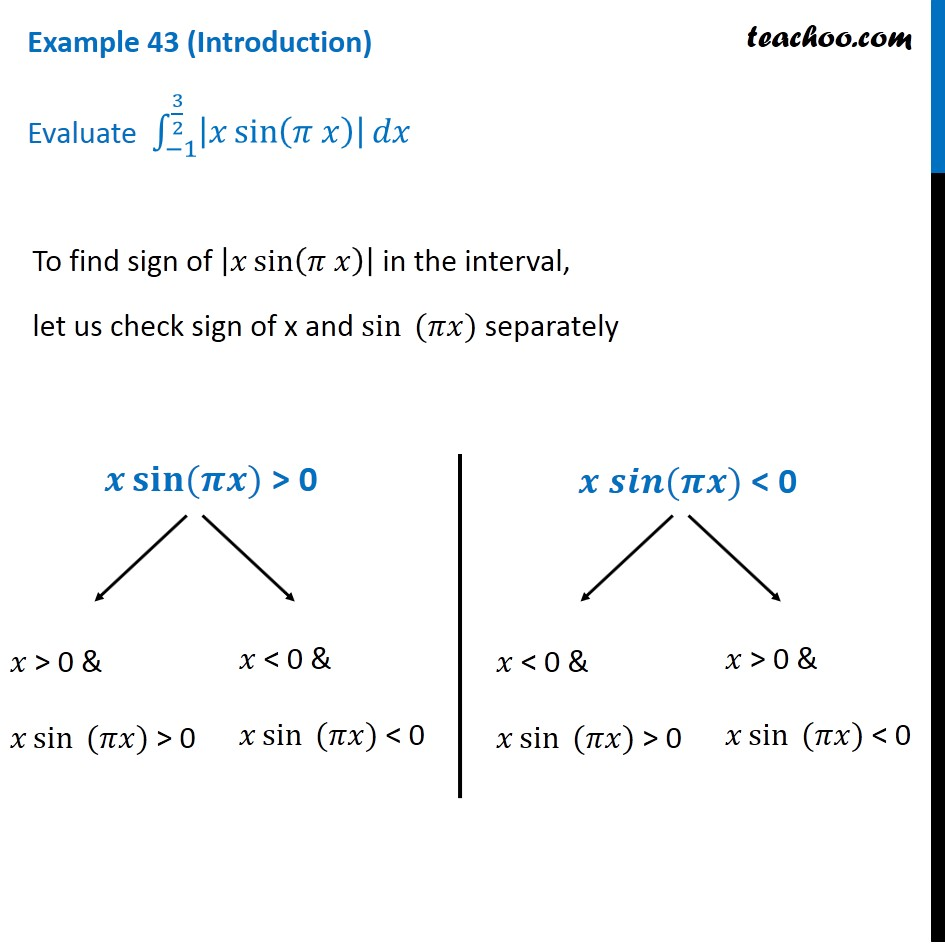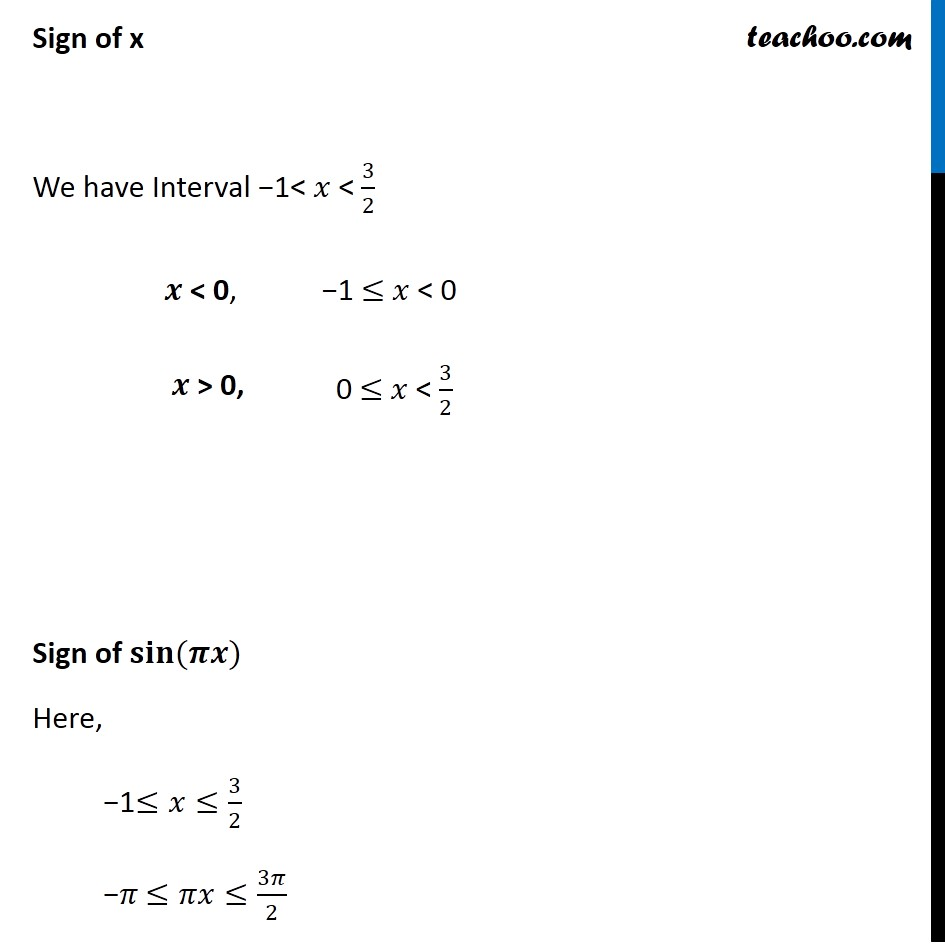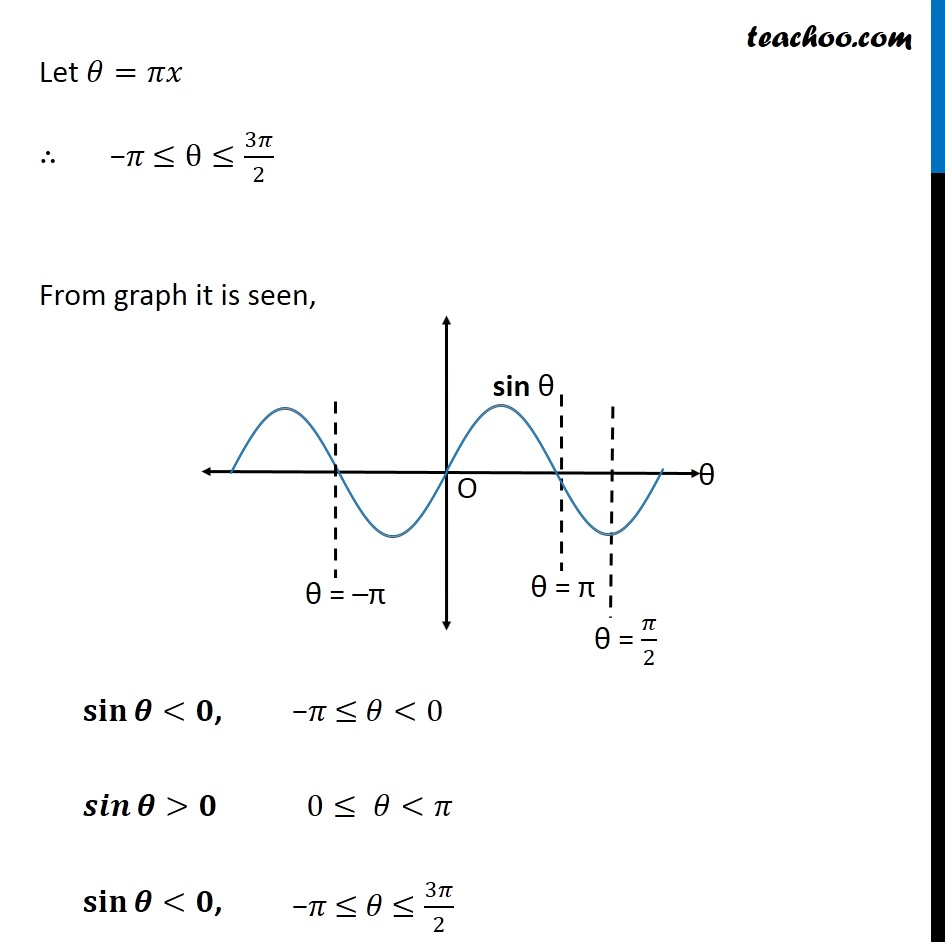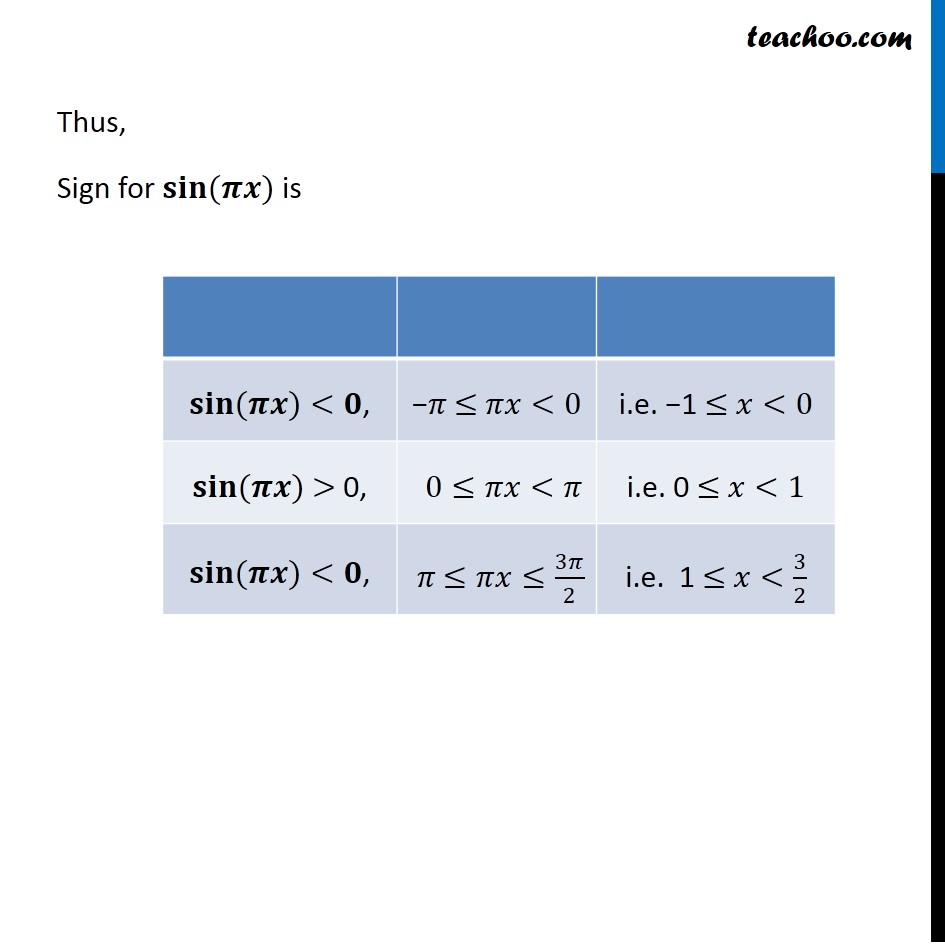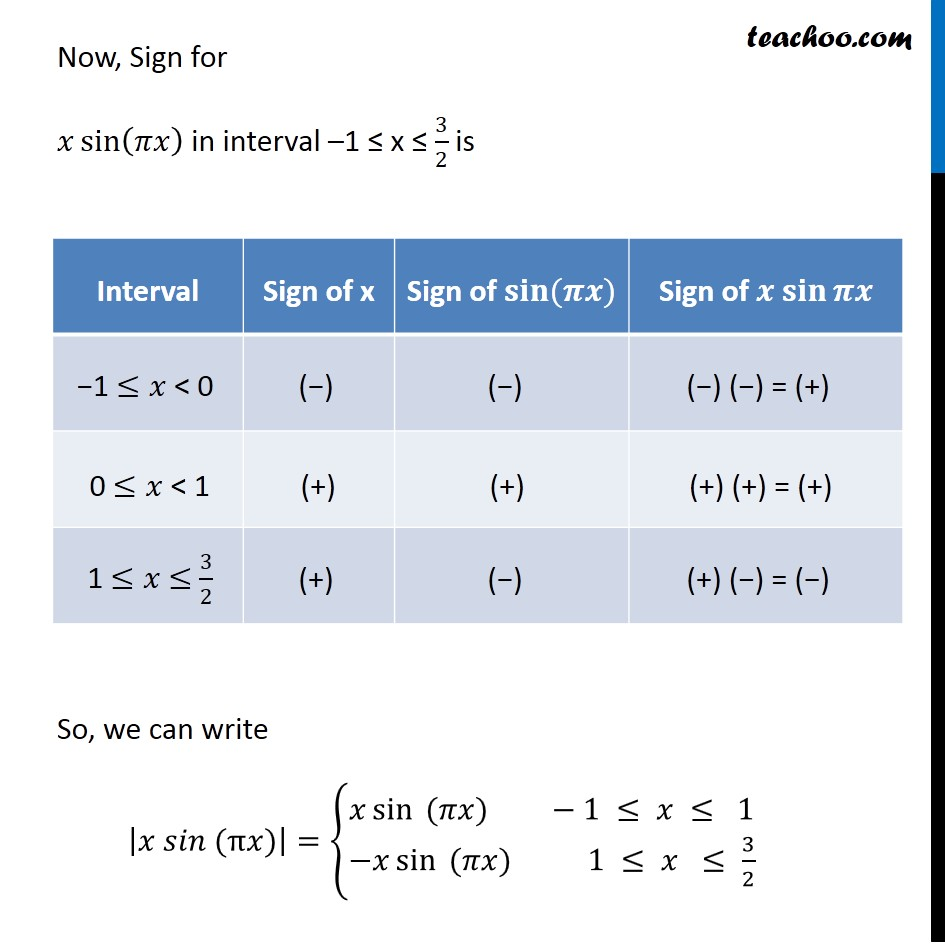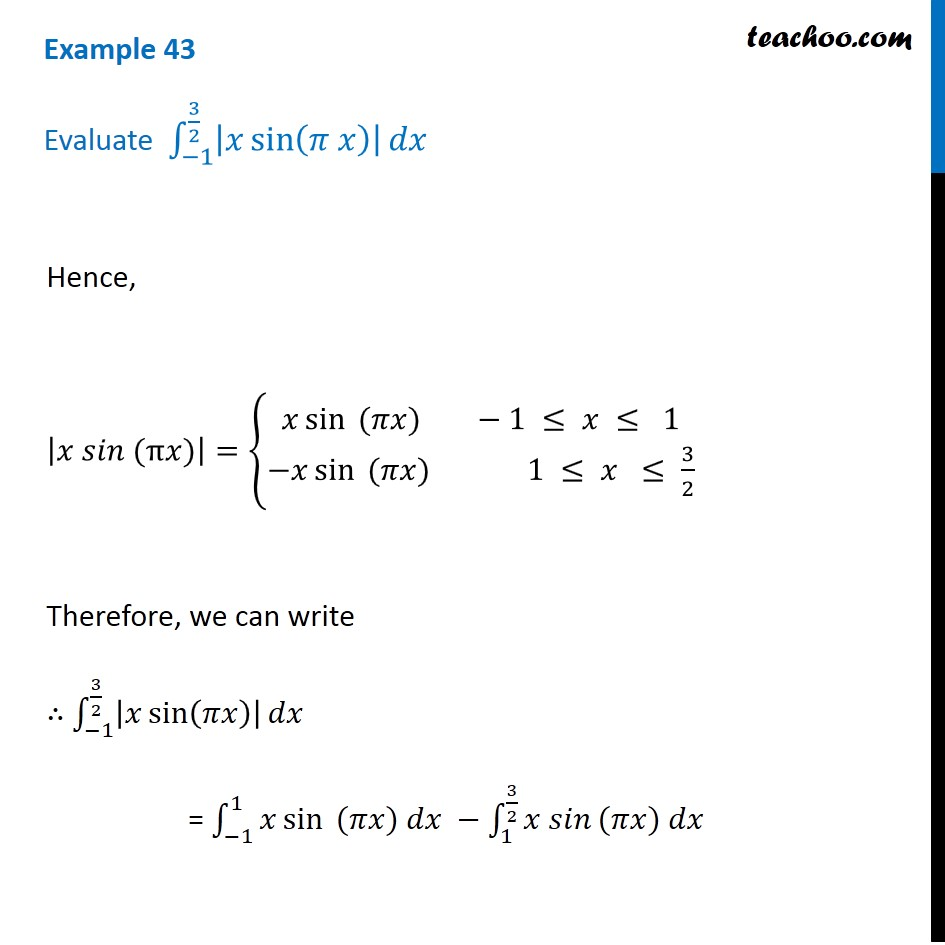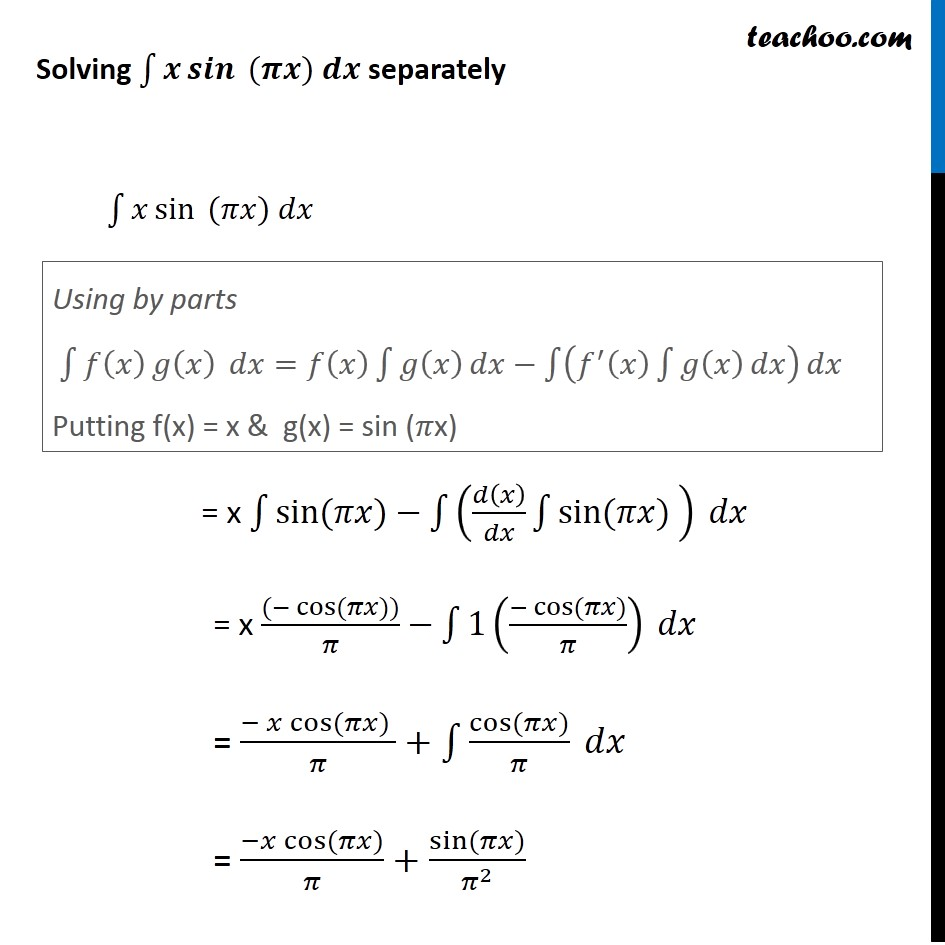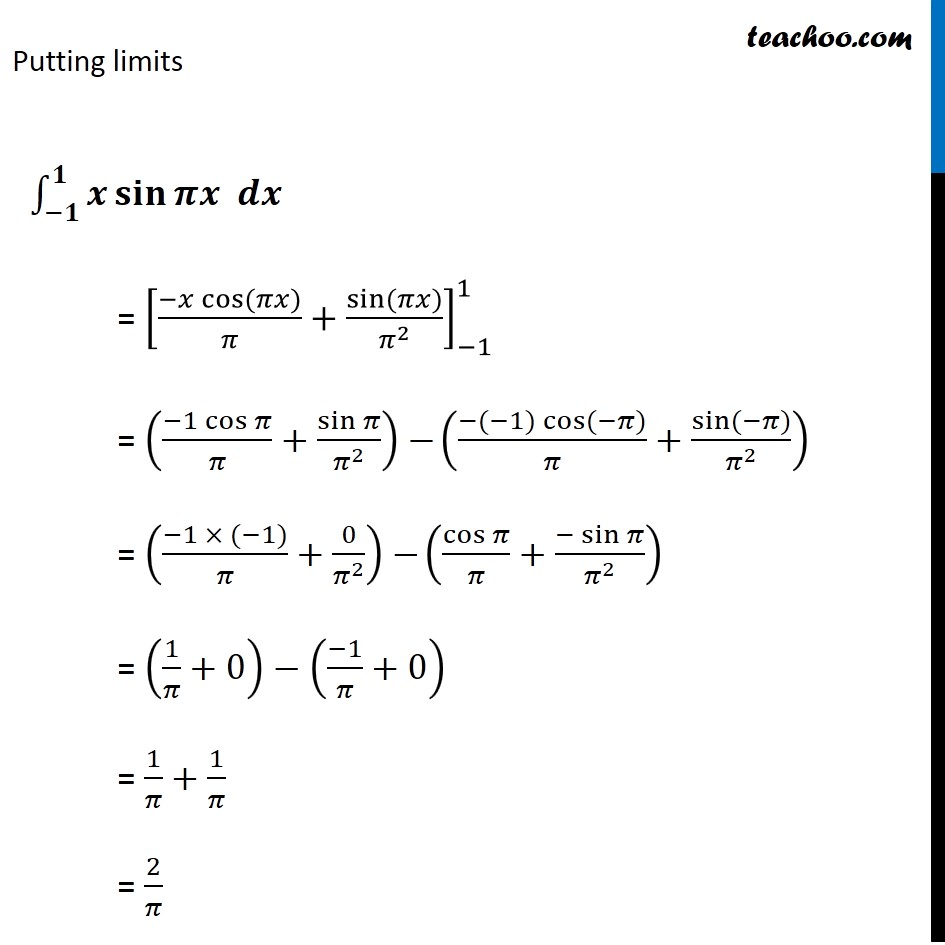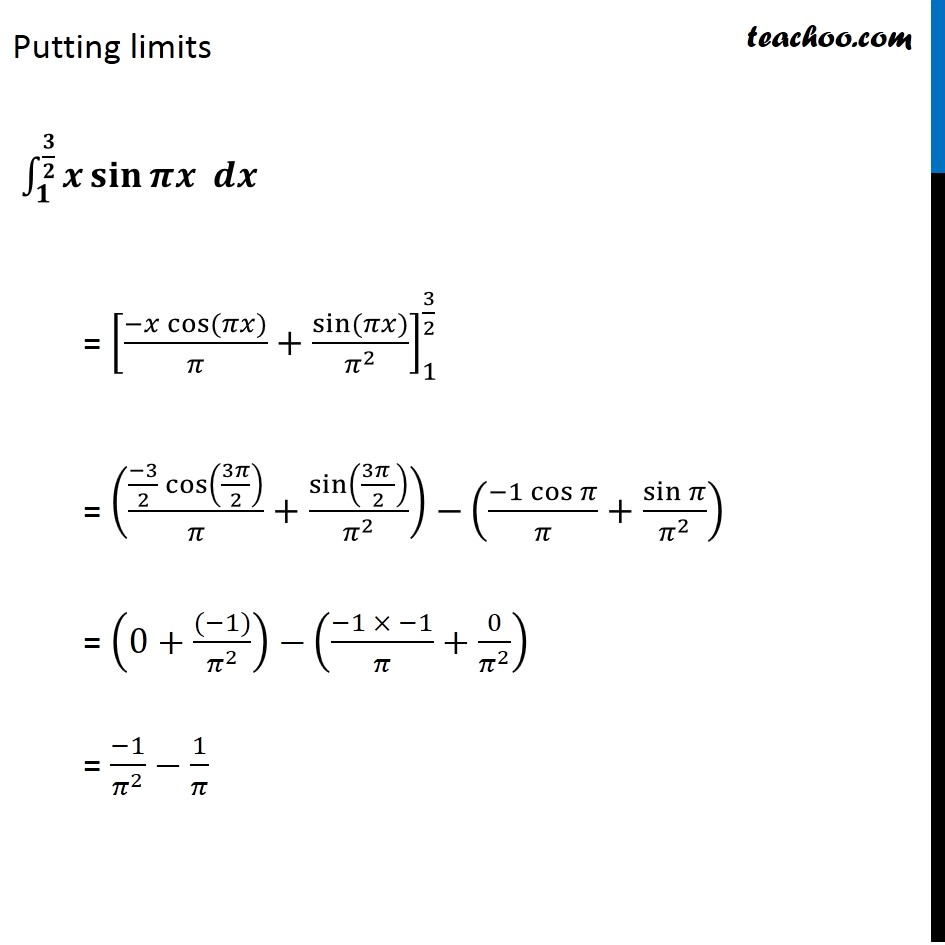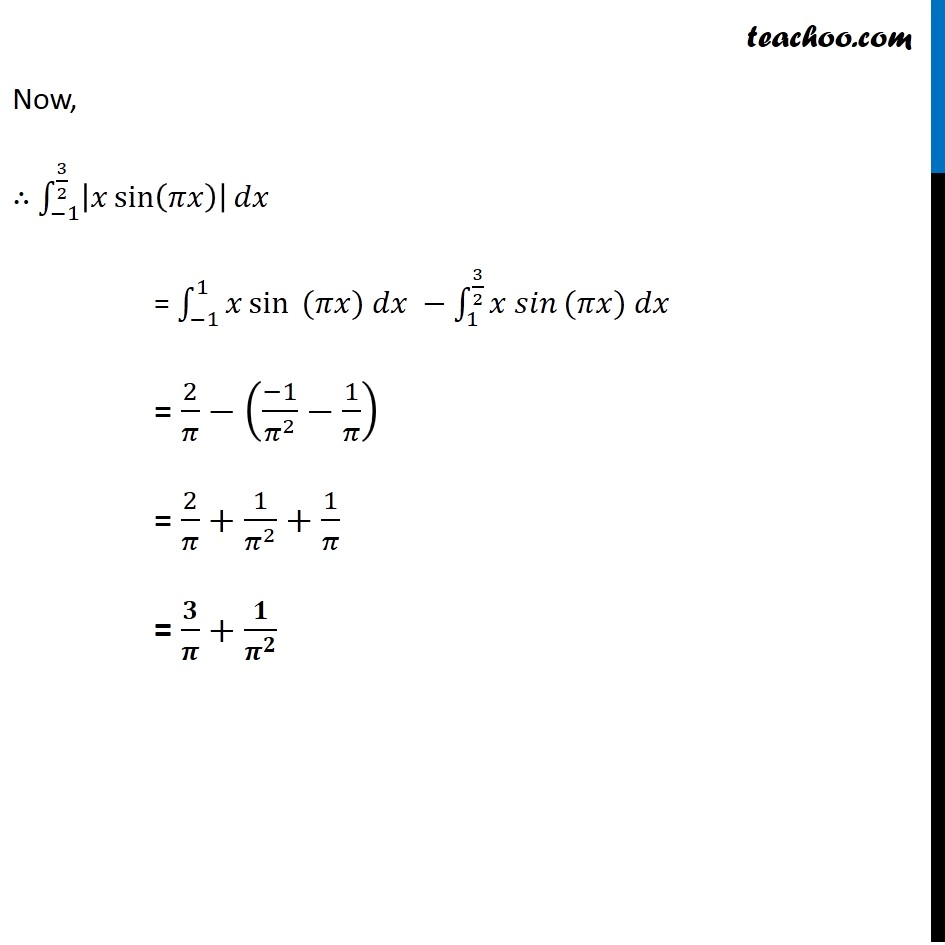1. Chapter 7 Class 12 Integrals
2. Concept wise
3. Definite Integration by properties - P2

Transcript

Example 43 (Introduction) Evaluate ∫_(−1)^(3/2)▒|𝑥 sin⁡(𝜋 𝑥) | 𝑑𝑥 To find sign of |𝑥 sin⁡(𝜋 𝑥) | in the interval, let us check sign of x and sin⁡〖 (𝜋𝑥) 〗separately 𝑥 > 0 & 𝑥 sin⁡〖 (𝜋𝑥) 〗> 0 𝑥 < 0 & 𝑥 sin⁡〖 (𝜋𝑥) 〗< 0 𝒙 𝒔𝒊𝒏⁡〖(𝝅𝒙) 〗< 0 𝑥 < 0 & 𝑥 sin⁡〖 (𝜋𝑥) 〗> 0 𝑥 > 0 & 𝑥 sin⁡〖 (𝜋𝑥) 〗< 0 Sign of x We have Interval −1< 𝑥 < 3/2 Sign of 𝐬𝐢𝐧⁡〖(𝝅𝒙)〗 Here, −1≤𝑥≤3/2 −𝜋 ≤𝜋𝑥≤3𝜋/2 Let 𝜃=𝜋𝑥 ∴ −𝜋 ≤θ≤3𝜋/2 From graph it is seen, Thus, Sign for 𝐬𝐢𝐧⁡〖(𝝅𝒙)〗 is Now, Sign for 𝑥 sin⁡(𝜋𝑥) in interval –1 ≤ x ≤ 3/2 is So, we can write |𝑥 𝑠𝑖𝑛 (π𝑥)|={█(𝑥 sin⁡〖 (𝜋𝑥)〗 −1 ≤ 𝑥 ≤ 1@&−𝑥 sin⁡〖 (𝜋𝑥)〗 1 ≤ 𝑥 ≤ 3/2)┤ Example 43 Evaluate ∫_(−1)^(3/2)▒|𝑥 sin⁡(𝜋 𝑥) | 𝑑𝑥 Hence, |𝑥 𝑠𝑖𝑛 (π𝑥)|={█(𝑥 sin⁡〖 (𝜋𝑥)〗 −1 ≤ 𝑥 ≤ 1@&−𝑥 sin⁡〖 (𝜋𝑥)〗 1 ≤ 𝑥 ≤ 3/2)┤ Therefore, we can write ∴ ∫_(−1)^(3/2)▒|𝑥 sin⁡(𝜋𝑥) | 𝑑𝑥 = ∫_(−1)^1▒〖𝑥 sin⁡〖 (𝜋𝑥) 𝑑𝑥 −∫_1^(3/2)▒〖𝑥 𝑠𝑖𝑛〗〗 〗(𝜋𝑥) 𝑑𝑥 Solving ∫1▒〖𝒙 𝒔𝒊𝒏⁡〖 (𝝅𝒙) 𝒅𝒙〗 〗 separately ∫1▒〖𝑥 sin⁡〖 (𝜋𝑥) 𝑑𝑥〗 〗 = x ∫1▒sin⁡〖(𝜋𝑥)−∫1▒(𝑑(𝑥)/𝑑𝑥 ∫1▒sin⁡〖(𝜋𝑥) 〗 ) 〗 𝑑𝑥 = x ((−cos⁡〖(𝜋𝑥))〗)/𝜋−∫1▒1((−cos⁡〖(𝜋𝑥)〗)/𝜋) 𝑑𝑥 = (− 𝑥 cos⁡〖(𝜋𝑥) 〗)/𝜋+∫1▒cos⁡〖(𝜋𝑥)〗/𝜋 𝑑𝑥 = (−𝑥 cos⁡〖(𝜋𝑥)〗)/𝜋+ sin⁡〖(𝜋𝑥)〗/𝜋^2 Using by parts ∫1▒〖𝑓(𝑥) 𝑔⁡(𝑥) 〗 𝑑𝑥=𝑓(𝑥) ∫1▒𝑔(𝑥) 𝑑𝑥−∫1▒(𝑓^′ (𝑥) ∫1▒𝑔(𝑥) 𝑑𝑥) 𝑑𝑥 Putting f(x) = x & g(x) = sin (𝜋x) Putting limits ∫_(−𝟏)^𝟏▒〖𝒙 𝐬𝐢𝐧⁡〖𝝅𝒙 𝒅𝒙〗 〗 = [(−𝑥 cos⁡〖(𝜋𝑥)〗)/𝜋+sin⁡〖(𝜋𝑥)〗/𝜋^2 ]_(−1)^1 = ((−1 cos⁡𝜋)/𝜋+sin⁡𝜋/𝜋^2 ) −((−(−1)cos⁡〖(−𝜋)〗)/𝜋+sin⁡〖(−𝜋)〗/𝜋^2 ) = ((−1 × (−1))/𝜋+0/𝜋^2 ) −(cos⁡𝜋/𝜋+〖− sin〗⁡𝜋/𝜋^2 ) = (1/𝜋+0)−((−1)/𝜋+0) = 1/𝜋+1/𝜋 = 2/𝜋 Putting limits ∫_𝟏^(𝟑/𝟐)▒〖𝒙 𝐬𝐢𝐧⁡〖𝝅𝒙 𝒅𝒙〗 〗 = [(−𝑥 cos⁡〖(𝜋𝑥)〗)/𝜋+sin⁡〖(𝜋𝑥)〗/𝜋^2 ]_1^(3/2) = (((−3)/2 cos⁡(3𝜋/2))/𝜋+sin⁡((3𝜋 )/2)/𝜋^2 )−((−1 cos⁡𝜋)/𝜋+sin⁡𝜋/𝜋^2 ) = (0+((−1))/𝜋^2 )−((−1 × −1)/𝜋+0/𝜋^2 ) = (−1)/𝜋^2 −1/𝜋 Now, ∴ ∫_(−1)^(3/2)▒|𝑥 sin⁡(𝜋𝑥) | 𝑑𝑥 = ∫_(−1)^1▒〖𝑥 sin⁡〖 (𝜋𝑥) 𝑑𝑥 −∫_1^(3/2)▒〖𝑥 𝑠𝑖𝑛〗〗 〗(𝜋𝑥) 𝑑𝑥 = 2/𝜋−((−1)/𝜋^2 −1/𝜋) = 2/𝜋+1/𝜋^2 +1/𝜋 = 𝟑/𝝅+𝟏/𝝅^𝟐

Definite Integration by properties - P2